#### Nodal and cuspidal curves

A point P of a curve f(x,y) = 0 is called nonsingular if the gradient f does not vanish at P. In this case, the implicit function theorem tells us that near P, y can be expressed as a function of x or the other way around. Most of the curves considered previously (parabola, circle elliptic curve) consist entirely of nonsingular points. The degenerate conic consisting of two nonparallel lines has a singularity where they meet. It is easy to construct singular cubics with several components as a union of a conic and a line, or three lines. The nodal cubic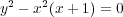has a singular point at the origin, and it is irreducible meaning that it is not a union of other curves. From an algebraic point of view, this amounts to the irreducibility of the defining polynomial. The singularity is clearly visible from the graph over reals. Here we see two tangent directions at the origin instead of just one.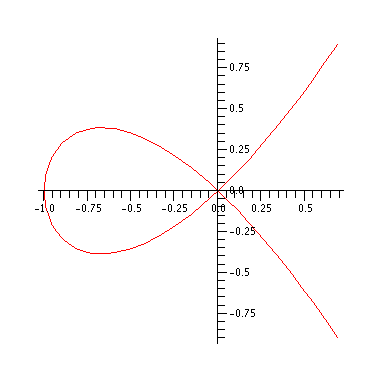In order to plot the complex graph, we reparameterize it by blowing up the singularity. This means that we seperate the lines at the origin, by introducing a new variable t = y∕x representing the slope of each line. Plotting this in x,y,z space, with x = t , yields the so called resolution of singularities (in black) of the original curve (in red).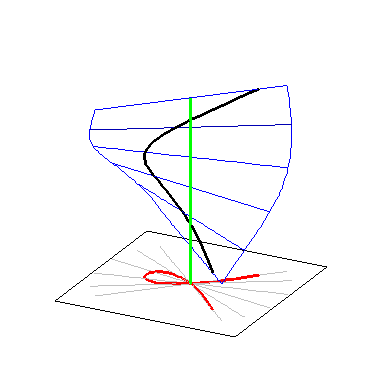We can find an explicit parameterization by substituting y = xt intowhich yields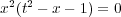This has two components corresponding to the factorization. These are given by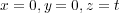depicted in green, and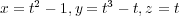in black. The first two equations give a rational parameterization of the the original curve. As we saw the elliptic curve considered above cannot admit such a parametrization. Thus inspite of the singularity, the present example is simpler. Setting t = r + is, with r and t real variables, and letting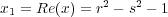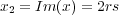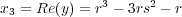and encoding Im(y) as the colour leads to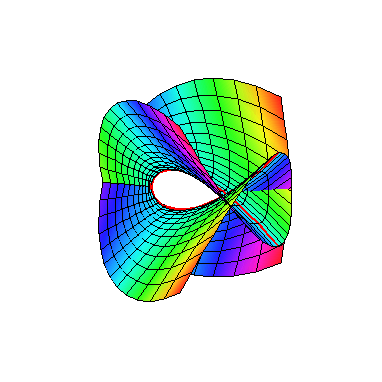Click on picture to start animation.
The real curve is marked in red. Notice that the graph looks similar to the graph of the elliptic curve above. This is because the nodal cubic can be viewed as limit of elliptic curves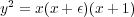as ε 0. In the process, the yellow curve in the previous graph -- called a vanishing cycle in this context -- shrinks to a point. So the global topology is different; it's no longer a torus. In fact, it's not difficult to see from the above parameterization that after adding a point at infinity, we get a sphere with the two points, t = 1 and t = -1, pinched together.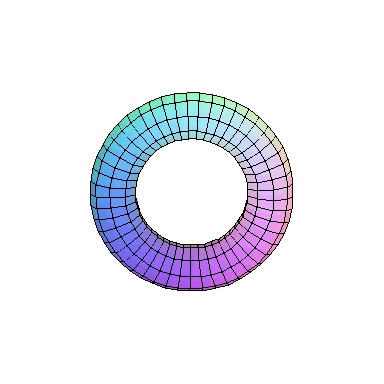We next look at another singular example called a (higher order) cusp y2 = x5. It is easy to see that x = t2, y = t5 gives a rational parameterization. This can arrived at by carrying out a resolution proceedure as above, but this time with two blow ups. Switching to polar coordinates (for purely aesthetic reasons) and taking real and imaginary parts as above, leads to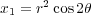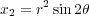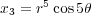This yields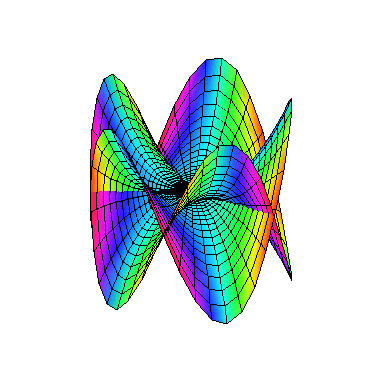Now take a look at the outer edge of this picture. The way it is drawn, it repeatedly crosses itself. But we know this really represents a defect of our depiction more than anything else. To get a better feeling for what this ought to look like, form the intersection of our cusp with a small sphere x2 + y2 = ε 2. This is called the link of the singularity. The sphere can be identified with R3 plus a point at infinity, and the link is a knot in this space. In this case, the link is a so called (2,5)-torus knot which looks as follows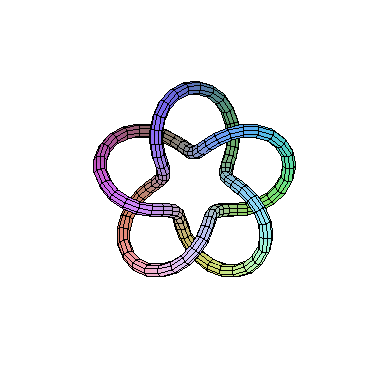If we did this for a nonsingular point, we would have gotten an unknotted circle. So the amount of "knottedness" is really telling us how bad the singularity is.